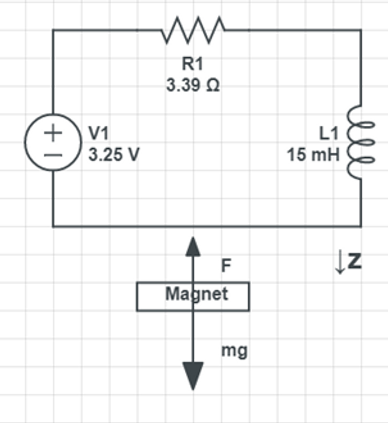## MagLev

The project goal is to control the voltage in the magnetic coil in order to levitate the magnet in the air.

The steps involves in this project including, dynamic system modeling, controller design and simulation, and hardware implementation.## Physical System

The physical system includes an inductor coil, a magnet, a Hall Effect Sensor, and an Arduino. The hall effect sensor is an analog sensor that could be used for measuring the distance. The distance feeds back to Arduino to regulate the output voltage to the inductor coil in order to levitate the magnet.## Mathematical Model

Subsystem

01

#### Electrical circuitSubsystem

02

#### Newton's LawSubsystem

03

#### Magnetic Force## State Variables and State Space

The state variables are current, magnet position, and magnet velocity.

The input is voltage.The state space equations can be calculated by combining all three subsystem equations and the state variables.## Model VerificationAfter I get the mathematical model of the mag lev, I coded it into a mass matrix form in MATLAB and simulated using ODE45 solver.

Above is the simulated results with 0V and 1V input voltages. The initial condition for this simulation is placing the magnet at 2 cm (0.02 m) away from the magnetic coil. When there is no voltage applied (0V input voltage), the magnet exhibits a free fall behavior. When there is a constant 1 volt input applied, the magnet sticks right to the coil, hence the position goes to zero. The position is the distance between the magnet and the magnetic coil.

This plot proves that my mathematical model works!

## Compensator Design

The mag lev is a nonlinear system. I first linearized the system around its equilibriums. Then I converted that linearized state space into a transfer function, which shows the relationship between the distance and the input voltage.There are 2 LHP poles and 1 RHP pole in the above system transfer function. The 1 RHP pole will cause system instable. In order to solve that, I designed a lead compensator to achieve stability.

I choose lead compensator is because it can decrease the rise time and settling time of the system. A fast response is extremely crucial in a mag lev system, since everything happens in a split of a second.

The root locus plot below shows the system behavior without compensator. The two conjugate roots are located in a way such that no matter where you place the gain, the system will be unstable.The root locus plot below is the system with the lead compensator. The gain can now be placed on the LHP, thus achieving a stable system.## Model Simulation

After the system model and compensator are defined, it is time to see if the system will behave as predicted. Everything can be made in a Simulink block diagram.The plot below is the simulated results. The rise and settling time was extremely fast. Little to none damping was observed. The next step is to implement it on the real system!## Hardware Implementation

The communication between Arduino and MATLAB is done using this package, written by Dr. Hurst.The plots below show the experiment results. The magnet was able to levitate at 0.02 m away from the coil in less than 0.1 seconds. This behavior is similar to what I predicted using simulation.## Demonstration